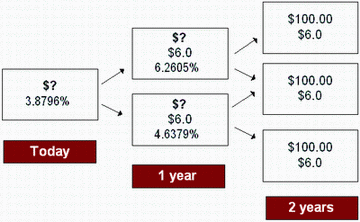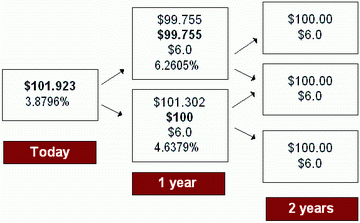### CFA Practice Question

There are 252 practice questions for this study session.

### CFA Practice Question

What is the value of a 2-year, 6% coupon, callable bond (in one year at 100) given the following interest rate tree?Current value of the bond at node 0 = [(99.755 + 6) / (1.038796) + (100.000 + 6) / (1.038796)] / 2 = 101.923

User Comment
katybo finally Im understanding binomial trees!
xyzanand Life is a binomial tree...
octavianus How do you calculate the call values in general? In this case, how do you determine \$99.755 (Up) and \$100 (down) for year 1 call values?

mchu Value at the Node = Min {Value from backward induction, Call price}

Call price is par.
tabulator octavianus, take some time reading notes and not jumping straigh to problems